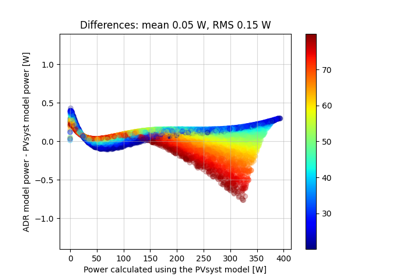# pvlib.pvsystem.max_power_point#

pvlib.pvsystem.max_power_point(photocurrent, saturation_current, resistance_series, resistance_shunt, nNsVth, d2mutau=0, NsVbi=inf, method='brentq')[source]#

Given the single diode equation coefficients, calculates the maximum power point (MPP).

Parameters
• photocurrent (numeric) – photo-generated current [A]

• saturation_current (numeric) – diode reverse saturation current [A]

• resistance_series (numeric) – series resitance [ohms]

• resistance_shunt (numeric) – shunt resitance [ohms]

• nNsVth (numeric) – product of thermal voltage Vth [V], diode ideality factor n, and number of serices cells Ns

• d2mutau (numeric, default 0) – PVsyst parameter for cadmium-telluride (CdTe) and amorphous-silicon (a-Si) modules that accounts for recombination current in the intrinsic layer. The value is the ratio of intrinsic layer thickness squared $$d^2$$ to the diffusion length of charge carriers $$\mu \tau$$. [V]

• NsVbi (numeric, default np.inf) – PVsyst parameter for cadmium-telluride (CdTe) and amorphous-silicon (a-Si) modules that is the product of the PV module number of series cells Ns and the builtin voltage Vbi of the intrinsic layer. [V].

• method (str) – either 'newton' or 'brentq'

Returns

OrderedDict or pandas.DataFrame(i_mp, v_mp, p_mp)

Notes

Use this function when you only want to find the maximum power point. Use singlediode() when you need to find additional points on the IV curve. This function uses Brent’s method by default because it is guaranteed to converge.

## Examples using pvlib.pvsystem.max_power_point#Fast simulation using the ADR efficiency model starting from PVsyst parameters

Fast simulation using the ADR efficiency model starting from PVsyst parameters